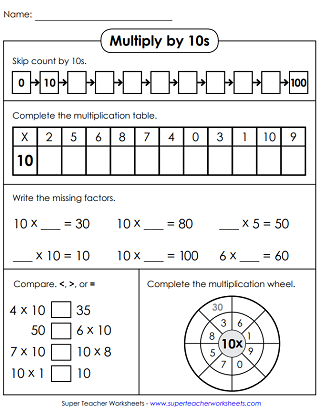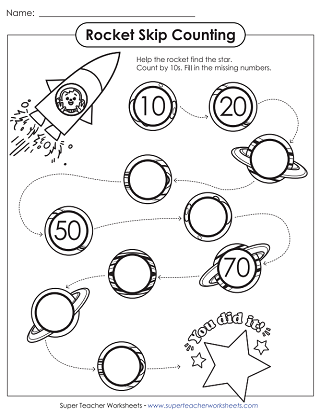# Multiplication by 10s (10s Facts Only)

With these printable worksheets, students can practice multiplying by the number 10. Includes all basic facts, up to 10x10, in which at least one factor is 10.

If you're looking for worksheets with all facts from 0-10, please jump to our Basic Multiplication 0-10 page.## Multiply By10s Only

This worksheet has a skip counting activity, a fill-in-the-blank multiplication table, multiplication wheel, and more.
This worksheet can be split and used for two students. Each caterpillar has 10 segments with basic multiply-by-10 math problems to be solved.
Help students perfect their multiplication skills with these task cards, which will help them review multiplying by 10s.
Use scissors to cut out each of these puzzle pieces. Arrange them on a flat surface to match each multiplication fact with the correct answer.
Write the answers to the multiplication facts on the caterpillar.
Here's an origami fortune teller (cootie catcher) game. Cut, color, and fold to make a toy that teaches kids to multiply by 10s.
This file contains 11 flash cards with basic facts, 5 super challenge cards, a self-test worksheet, and a flash card sorting mat.
Cut and color the truck to make the multiplication slider craft. Then insert math sliders to practice basic facts with 10 as a factor.

## Skip CountBy 10s

Fill in the missing numbers on 3 numbers lines. (The main version of this worksheet goes up to 100. If you'd like a more advanced version that goes up to 180, click the alt button below.
Count by 10s and connect the dots. The finished picture is a pig.

## All Facts0 through 10s

All Multiplication Facts (0-10)

For all basic multiplication facts with factors between 0 and 10, please click the link above to visit our our main basic multiplication page.

Basic Multiplication Facts

This page has worksheets with 0-10 facts, 0-12 facts, arrays, fact families, and more.

Multiplying by 9s Only

This page has worksheets, task cards, puzzles, flash cards, and sliders with multiplication facts that have 9 as a factor.

Multiplication by 8s Only

Here you'll find a collection of printables with multiplication facts that have 8 as a factor.

Multiplication by 7s Only

Here are the worksheets for learning to multiply pairs of numbers with 7 as one of the factors.

## Sample Worksheet ImagesMy Account
Site Information